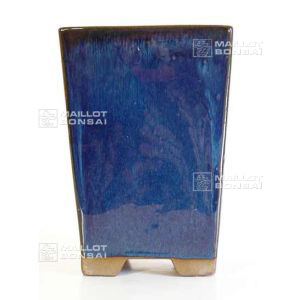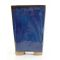##### The Japanese Bonsai specialist
Direct order Contact Help / Services Newsletter# 28 coloured glazed pot blue

› Bonsai pots › Cascade potsref. : 4791

27,50

Available quantity : 10Order

###### Description

Exterior: 12.5*12.5*18 cm. Interior: 11*11*15 cm. Colour: blue. Our small, tall pots come in a range of vibrant shades to add a colour pop to your home and garden. Line them up on your windowsill, create a mini-pot arrangement of sedums or cacti, or else plant a cascading 'kengai' bonsai or semi-cascade 'han kengai' bonsai.

#cascade 3.7 #pots 3.4 #blue 3.4 #coloured 2.8 #glazed 2.6 #bonsai 2.6 #kengai 2.6 #colour 2.6 #your 2.4 #arrangement 2.1

Formule
(( ROUND((CHAR_LENGTH(b.article_nom)-CHAR_LENGTH(REPLACE(b.article_nom, 'blue', '')))/LENGTH('blue')) + ROUND((CHAR_LENGTH(b.article_description)-CHAR_LENGTH(REPLACE(b.article_description, 'blue', '')))/LENGTH('blue')) ) * 3.4) + (( ROUND((CHAR_LENGTH(b.article_nom)-CHAR_LENGTH(REPLACE(b.article_nom, 'coloured', '')))/LENGTH('coloured')) + ROUND((CHAR_LENGTH(b.article_description)-CHAR_LENGTH(REPLACE(b.article_description, 'coloured', '')))/LENGTH('coloured')) ) * 2.8) + (( ROUND((CHAR_LENGTH(b.article_nom)-CHAR_LENGTH(REPLACE(b.article_nom, 'bonsai', '')))/LENGTH('bonsai')) + ROUND((CHAR_LENGTH(b.article_description)-CHAR_LENGTH(REPLACE(b.article_description, 'bonsai', '')))/LENGTH('bonsai')) ) * 2.6) + (( ROUND((CHAR_LENGTH(b.article_nom)-CHAR_LENGTH(REPLACE(b.article_nom, 'kengai', '')))/LENGTH('kengai')) + ROUND((CHAR_LENGTH(b.article_description)-CHAR_LENGTH(REPLACE(b.article_description, 'kengai', '')))/LENGTH('kengai')) ) * 2.6) + (( ROUND((CHAR_LENGTH(b.article_nom)-CHAR_LENGTH(REPLACE(b.article_nom, 'glazed', '')))/LENGTH('glazed')) + ROUND((CHAR_LENGTH(b.article_description)-CHAR_LENGTH(REPLACE(b.article_description, 'glazed', '')))/LENGTH('glazed')) ) * 2.6) + (( ROUND((CHAR_LENGTH(b.article_nom)-CHAR_LENGTH(REPLACE(b.article_nom, 'colour', '')))/LENGTH('colour')) + ROUND((CHAR_LENGTH(b.article_description)-CHAR_LENGTH(REPLACE(b.article_description, 'colour', '')))/LENGTH('colour')) ) * 2.6) + (( ROUND((CHAR_LENGTH(b.article_nom)-CHAR_LENGTH(REPLACE(b.article_nom, 'your', '')))/LENGTH('your')) + ROUND((CHAR_LENGTH(b.article_description)-CHAR_LENGTH(REPLACE(b.article_description, 'your', '')))/LENGTH('your')) ) * 2.4) + (( ROUND((CHAR_LENGTH(b.article_nom)-CHAR_LENGTH(REPLACE(b.article_nom, 'arrangement', '')))/LENGTH('arrangement')) + ROUND((CHAR_LENGTH(b.article_description)-CHAR_LENGTH(REPLACE(b.article_description, 'arrangement', '')))/LENGTH('arrangement')) ) * 2.1) + (( ROUND((CHAR_LENGTH(b.article_nom)-CHAR_LENGTH(REPLACE(b.article_nom, 'windowsill', '')))/LENGTH('windowsill')) + ROUND((CHAR_LENGTH(b.article_description)-CHAR_LENGTH(REPLACE(b.article_description, 'windowsill', '')))/LENGTH('windowsill')) ) * 2) + (( ROUND((CHAR_LENGTH(b.article_nom)-CHAR_LENGTH(REPLACE(b.article_nom, 'cascading', '')))/LENGTH('cascading')) + ROUND((CHAR_LENGTH(b.article_description)-CHAR_LENGTH(REPLACE(b.article_description, 'cascading', '')))/LENGTH('cascading')) ) * 1.9)

## Secure payment## Delivery

Our logistic partners :